# Copyright 2016 2012 Pearson Education Inc 2 2

• Slides: 39
Download presentation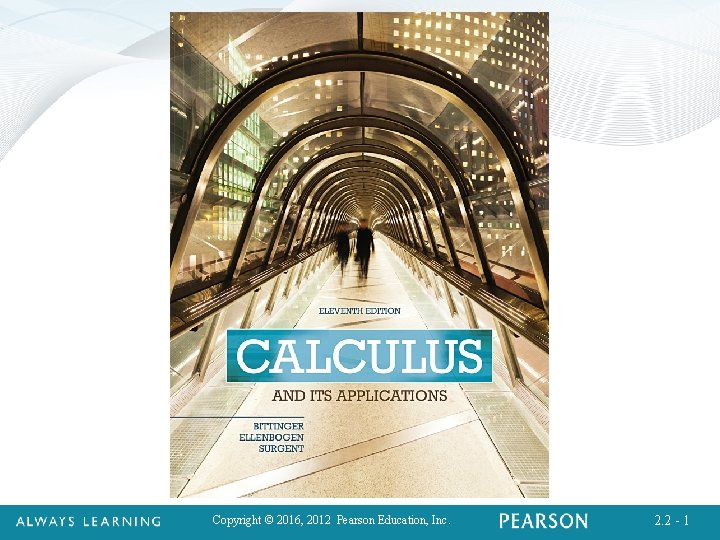Copyright © 2016, 2012 Pearson Education, Inc. 2. 2 - 1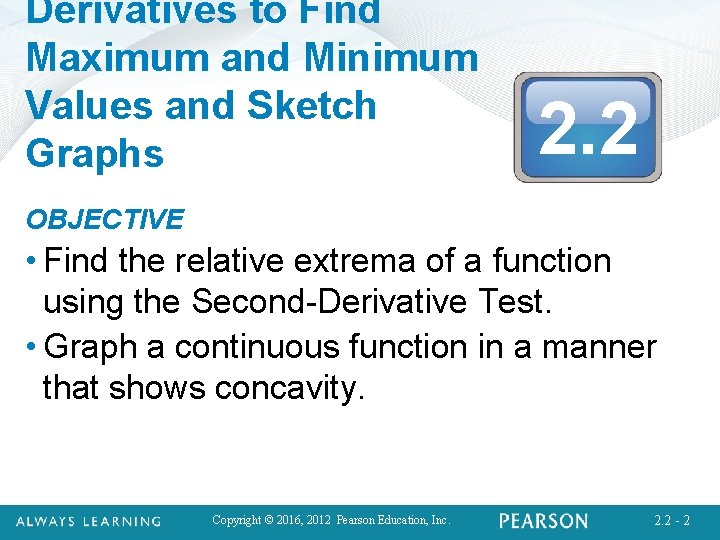Derivatives to Find Maximum and Minimum Values and Sketch Graphs 2. 2 OBJECTIVE • Find the relative extrema of a function using the Second-Derivative Test. • Graph a continuous function in a manner that shows concavity. Copyright © 2016, 2012 Pearson Education, Inc. 2. 2 - 2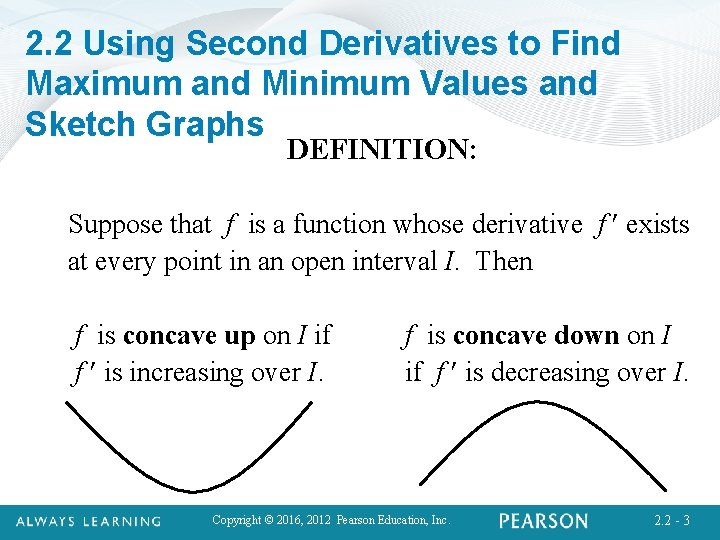2. 2 Using Second Derivatives to Find Maximum and Minimum Values and Sketch Graphs DEFINITION: Suppose that f is a function whose derivative f exists at every point in an open interval I. Then f is concave up on I if f is increasing over I. f is concave down on I if f is decreasing over I. Copyright © 2016, 2012 Pearson Education, Inc. 2. 2 - 32. 2 Using Second Derivatives to Find Maximum and Minimum Values and Sketch Graphs THEOREM 4: A Test for Concavity 1. If f (x) > 0 on an interval I, then the graph of f is concave up. ( f is increasing, so f is turning up on I. ) 2. If f (x) < 0 on an interval I, then the graph of f is concave down. ( f is decreasing, so f is turning down on I. ) Copyright © 2016, 2012 Pearson Education, Inc. 2. 2 - 42. 2 Using Second Derivatives to Find Maximum and Minimum Values and Sketch Graphs THEOREM 5: The Second Derivative Test for Relative Extrema Suppose that f is differentiable for every x in an open interval (a, b) and that there is a critical value c in (a, b) for which f (c) = 0. Then: 1. f (c) is a relative minimum if f (c) > 0. 2. f (c) is a relative maximum if f (c) < 0. For f (c) = 0, the First-Derivative Test can be used to determine whether f (c) is a relative extremum. Copyright © 2016, 2012 Pearson Education, Inc. 2. 2 - 5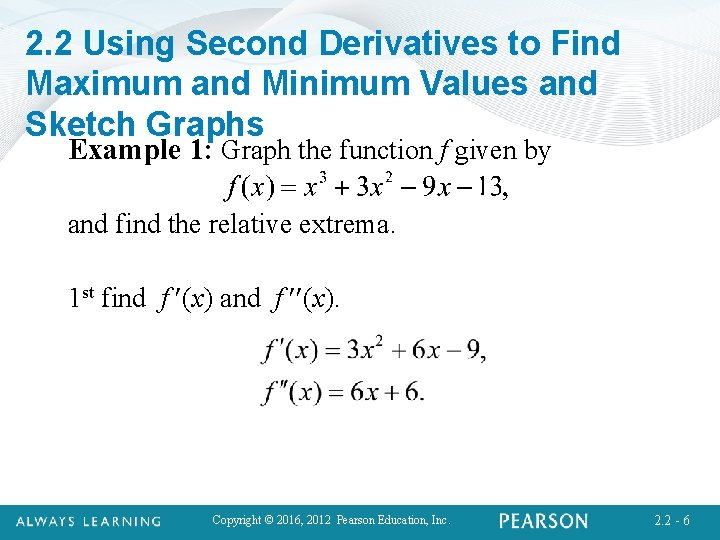2. 2 Using Second Derivatives to Find Maximum and Minimum Values and Sketch Graphs Example 1: Graph the function f given by and find the relative extrema. 1 st find f (x) and f (x). Copyright © 2016, 2012 Pearson Education, Inc. 2. 2 - 62. 2 Using Second Derivatives to Find Maximum and Minimum Values and Sketch Graphs Example 1 (continued): 2 nd solve f (x) = 0. Thus, x = – 3 and x = 1 are critical values. Copyright © 2016, 2012 Pearson Education, Inc. 2. 2 - 7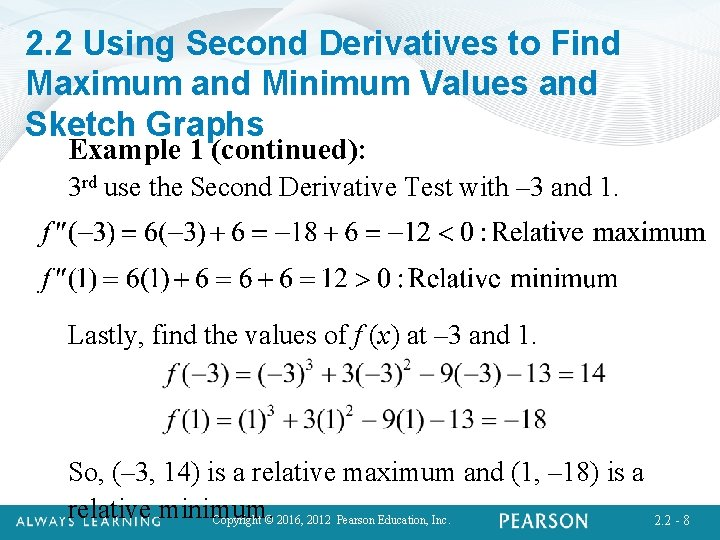2. 2 Using Second Derivatives to Find Maximum and Minimum Values and Sketch Graphs Example 1 (continued): 3 rd use the Second Derivative Test with – 3 and 1. Lastly, find the values of f (x) at – 3 and 1. So, (– 3, 14) is a relative maximum and (1, – 18) is a relative minimum. Copyright © 2016, 2012 Pearson Education, Inc. 2. 2 - 8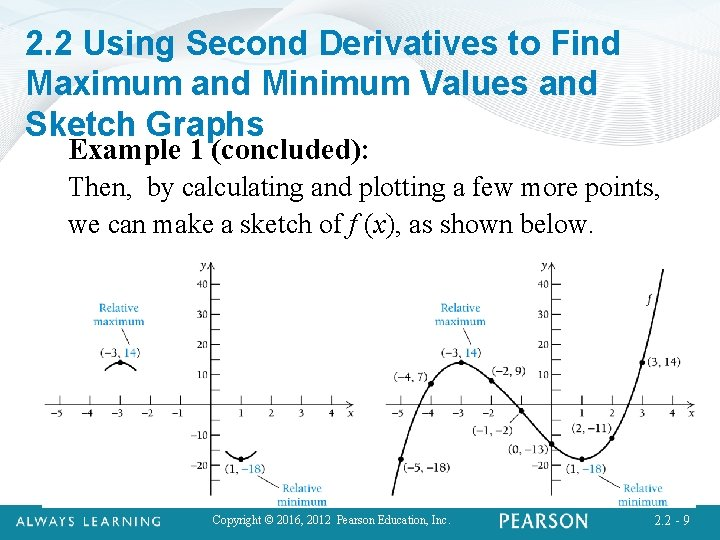2. 2 Using Second Derivatives to Find Maximum and Minimum Values and Sketch Graphs Example 1 (concluded): Then, by calculating and plotting a few more points, we can make a sketch of f (x), as shown below. Copyright © 2016, 2012 Pearson Education, Inc. 2. 2 - 92. 2 Using Second Derivatives to Find Maximum and Minimum Values and Sketch Graphs Quick Check 1 Find the relative extrema of the function and sketch the graph. First find and given by , : Copyright © 2016, 2012 Pearson Education, Inc. 2. 2 - 10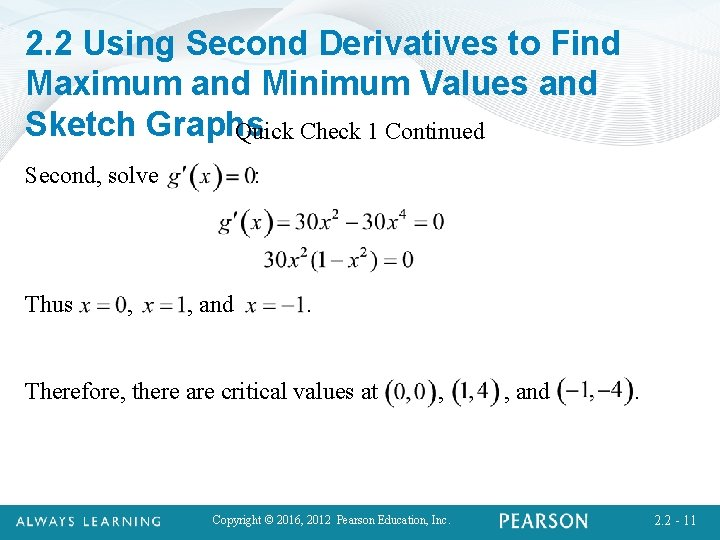2. 2 Using Second Derivatives to Find Maximum and Minimum Values and Sketch Graphs Quick Check 1 Continued Second, solve Thus , : , and . Therefore, there are critical values at , Copyright © 2016, 2012 Pearson Education, Inc. , and . 2. 2 - 11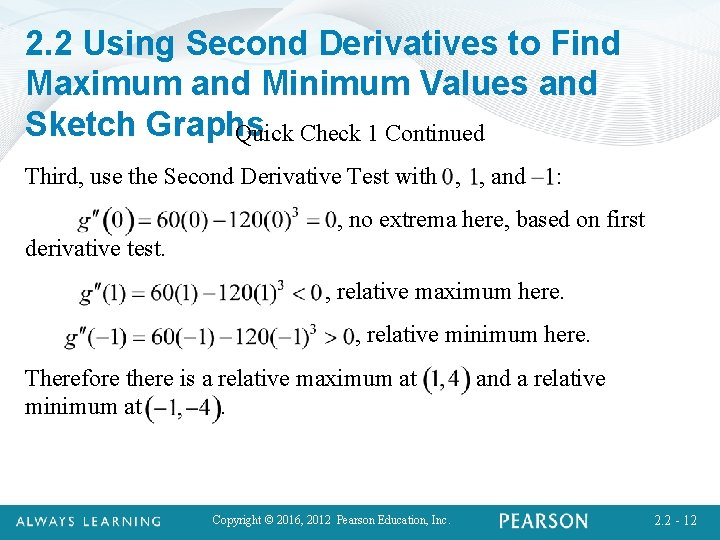2. 2 Using Second Derivatives to Find Maximum and Minimum Values and Sketch Graphs Quick Check 1 Continued Third, use the Second Derivative Test with , , and : , no extrema here, based on first derivative test. , relative maximum here. , relative minimum here. Therefore there is a relative maximum at minimum at. Copyright © 2016, 2012 Pearson Education, Inc. and a relative 2. 2 - 12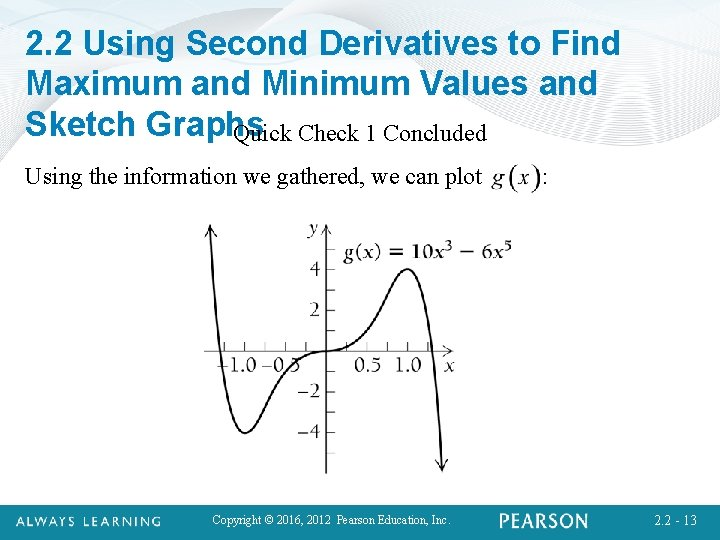2. 2 Using Second Derivatives to Find Maximum and Minimum Values and Sketch Graphs Quick Check 1 Concluded Using the information we gathered, we can plot Copyright © 2016, 2012 Pearson Education, Inc. : 2. 2 - 13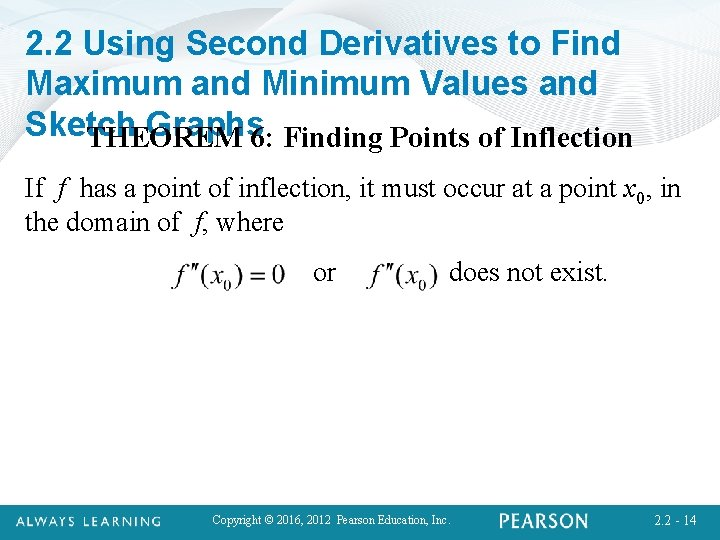2. 2 Using Second Derivatives to Find Maximum and Minimum Values and Sketch Graphs 6: Finding Points of Inflection THEOREM If f has a point of inflection, it must occur at a point x 0, in the domain of f, where or does not exist. Copyright © 2016, 2012 Pearson Education, Inc. 2. 2 - 14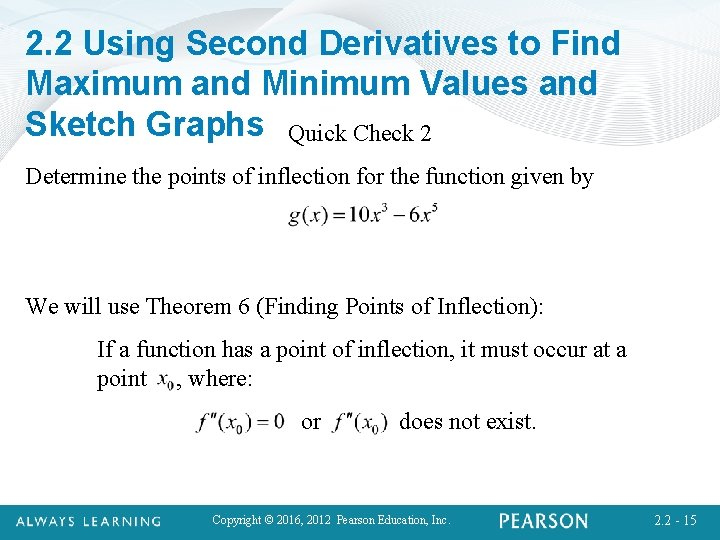2. 2 Using Second Derivatives to Find Maximum and Minimum Values and Sketch Graphs Quick Check 2 Determine the points of inflection for the function given by We will use Theorem 6 (Finding Points of Inflection): If a function has a point of inflection, it must occur at a point , where: or does not exist. Copyright © 2016, 2012 Pearson Education, Inc. 2. 2 - 15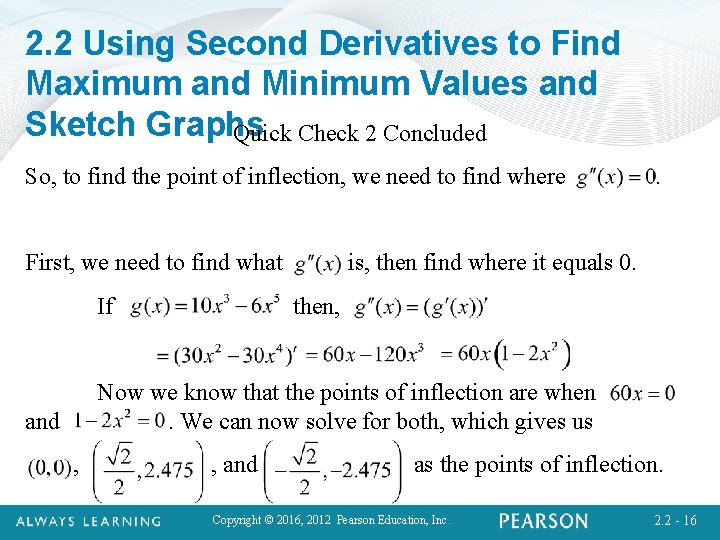2. 2 Using Second Derivatives to Find Maximum and Minimum Values and Sketch Graphs Quick Check 2 Concluded So, to find the point of inflection, we need to find where First, we need to find what If . is, then find where it equals 0. then, Now we know that the points of inflection are when. We can now solve for both, which gives us and , , and as the points of inflection. Copyright © 2016, 2012 Pearson Education, Inc. 2. 2 - 162. 2 Using Second Derivatives to Find Maximum and Minimum Values and Sketch Strategy. Graphs for Sketching Graphs: a) Derivatives and Domain. Find f (x) and f (x). Note the domain of f. b) Critical values of f. Find the critical values by solving f (x) = 0 and finding where f (x) does not exist. These numbers yield candidates for relative maxima or minima. Find the function values at these points. c) Increasing and/or decreasing; relative extrema. Substitute each critical value, x 0, from step (b) into f (x). If f (x 0) < 0, then f (x 0) is a relative maximum Copyright © 2016, 2012 Pearson Education, Inc. 2. 2 - 172. 2 Using Second Derivatives to Find Maximum and Minimum Values and Sketch Strategy. Graphs for Sketching Graphs (continued): and f is increasing on the left of x 0 and decreasing on the right. If f (x 0) > 0, then f (x 0) is a relative minimum and f is decreasing on the left of x 0 and increasing on the right. d) Inflection Points. Determine candidates for inflection points by finding where f (x) = 0 or where f (x) does not exist. Find the function values at these points. Copyright © 2016, 2012 Pearson Education, Inc. 2. 2 - 18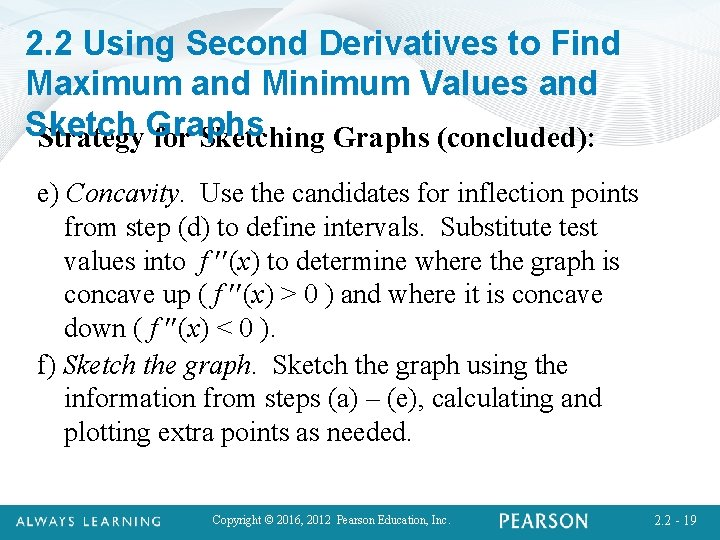2. 2 Using Second Derivatives to Find Maximum and Minimum Values and Sketch Strategy. Graphs for Sketching Graphs (concluded): e) Concavity. Use the candidates for inflection points from step (d) to define intervals. Substitute test values into f (x) to determine where the graph is concave up ( f (x) > 0 ) and where it is concave down ( f (x) < 0 ). f) Sketch the graph using the information from steps (a) – (e), calculating and plotting extra points as needed. Copyright © 2016, 2012 Pearson Education, Inc. 2. 2 - 19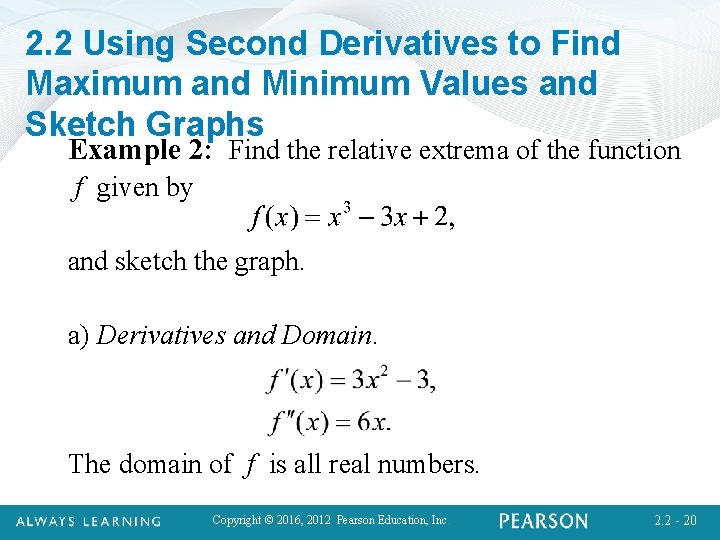2. 2 Using Second Derivatives to Find Maximum and Minimum Values and Sketch Graphs Example 2: Find the relative extrema of the function f given by and sketch the graph. a) Derivatives and Domain. The domain of f is all real numbers. Copyright © 2016, 2012 Pearson Education, Inc. 2. 2 - 20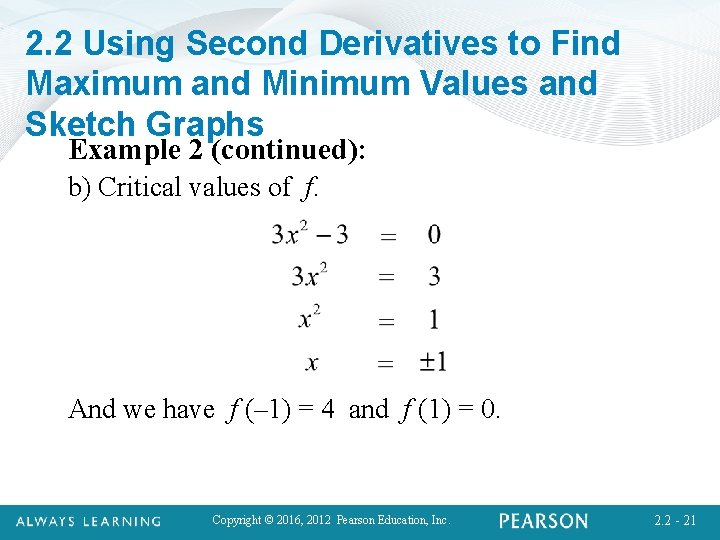2. 2 Using Second Derivatives to Find Maximum and Minimum Values and Sketch Graphs Example 2 (continued): b) Critical values of f. And we have f (– 1) = 4 and f (1) = 0. Copyright © 2016, 2012 Pearson Education, Inc. 2. 2 - 21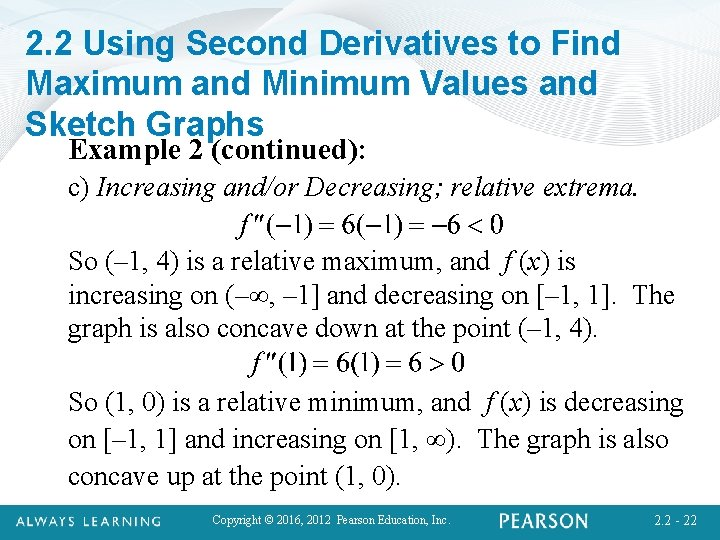2. 2 Using Second Derivatives to Find Maximum and Minimum Values and Sketch Graphs Example 2 (continued): c) Increasing and/or Decreasing; relative extrema. So (– 1, 4) is a relative maximum, and f (x) is increasing on (–∞, – 1] and decreasing on [– 1, 1]. The graph is also concave down at the point (– 1, 4). So (1, 0) is a relative minimum, and f (x) is decreasing on [– 1, 1] and increasing on [1, ∞). The graph is also concave up at the point (1, 0). Copyright © 2016, 2012 Pearson Education, Inc. 2. 2 - 222. 2 Using Second Derivatives to Find Maximum and Minimum Values and Sketch Graphs Example 2 (continued): d) Inflection Points. And we have f (0) = 2. e) Concavity. From step (c), we can conclude that f is concave down on the interval (–∞, 0) and concave up on (0, ∞). Copyright © 2016, 2012 Pearson Education, Inc. 2. 2 - 23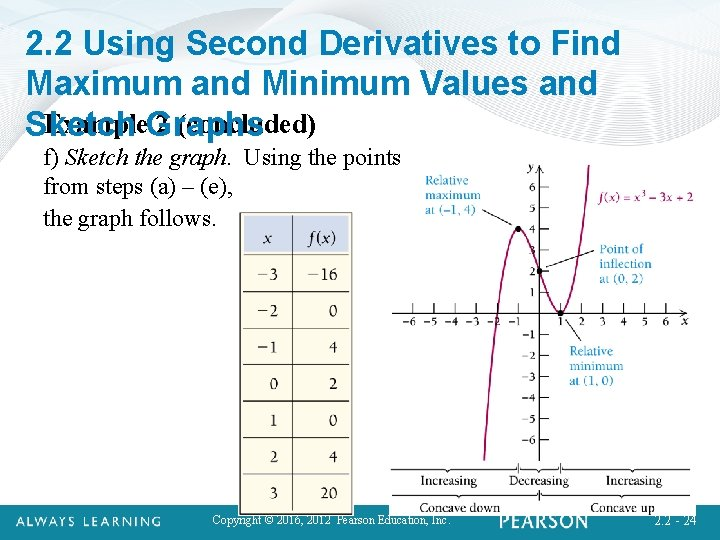2. 2 Using Second Derivatives to Find Maximum and Minimum Values and Example. Graphs 2 (concluded) Sketch f) Sketch the graph. Using the points from steps (a) – (e), the graph follows. Copyright © 2016, 2012 Pearson Education, Inc. 2. 2 - 242. 2 Using Second Derivatives to Find Maximum and Minimum Values and Sketch Graphs Quick Check 3 Find the relative maxima and minima of the function and sketch the graph. given by a. ) Derivatives and Domain The Domain of is all real numbers. Copyright © 2016, 2012 Pearson Education, Inc. 2. 2 - 25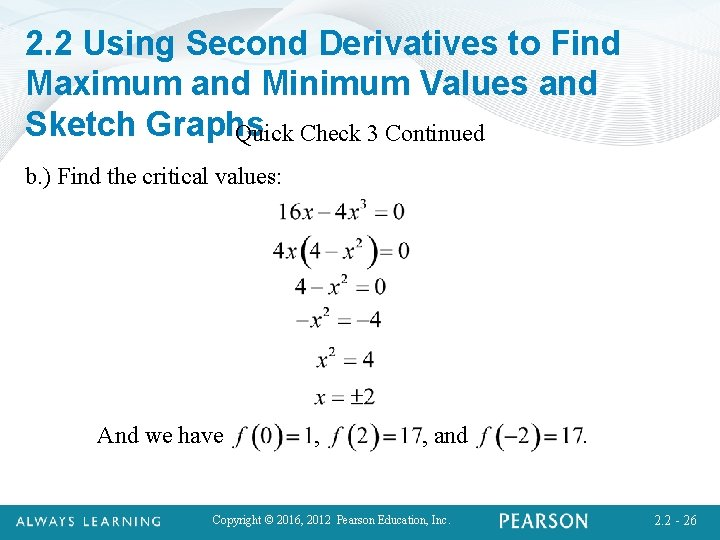2. 2 Using Second Derivatives to Find Maximum and Minimum Values and Sketch Graphs Quick Check 3 Continued b. ) Find the critical values: And we have , , and Copyright © 2016, 2012 Pearson Education, Inc. . 2. 2 - 262. 2 Using Second Derivatives to Find Maximum and Minimum Values and Quick Check 3 Continued Sketch Graphs c. ) Increasing and/or decreasing; relative extrema. So is a relative minimum, and is decreasing on increasing on. Also, the graph is concave up at. and So is a relative maximum, and is increasing on decreasing on. Also, the graph is concave down at and So is a relative maximum, and is increasing on and decreasing on. Also, the graph is concave down at Copyright © 2016, 2012 Pearson Education, Inc. . 2. 2 - 27 .2. 2 Using Second Derivatives to Find Maximum and Minimum Values and Sketch Graphs Quick Check 3 Continued d. ) Inflection Points. e. ) Concavity: From step c. ) we can conclude that is concave down on the intervals and , and is concave up on the interval. Copyright © 2016, 2012 Pearson Education, Inc. 2. 2 - 28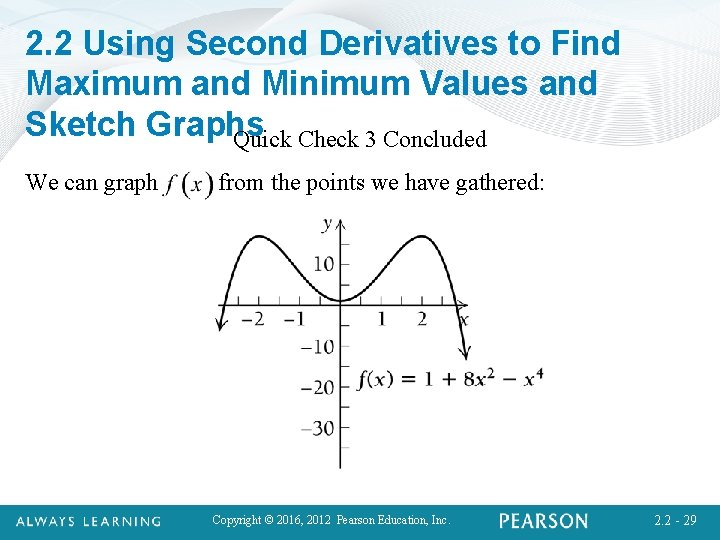2. 2 Using Second Derivatives to Find Maximum and Minimum Values and Sketch Graphs Quick Check 3 Concluded We can graph from the points we have gathered: Copyright © 2016, 2012 Pearson Education, Inc. 2. 2 - 29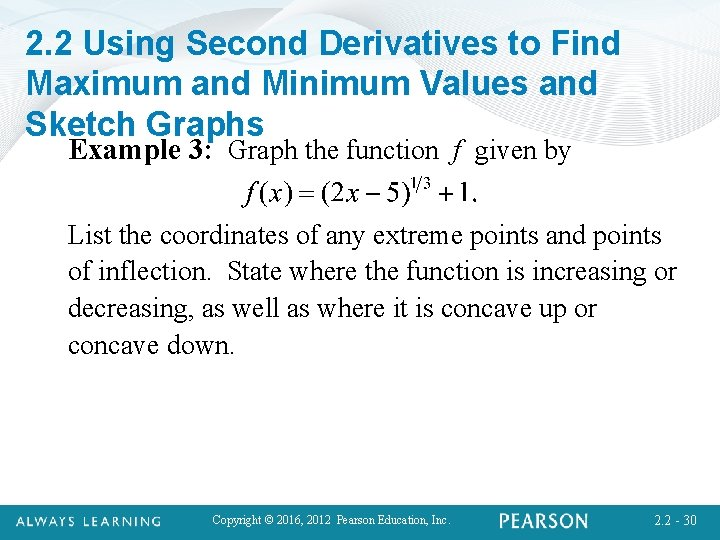2. 2 Using Second Derivatives to Find Maximum and Minimum Values and Sketch Graphs Example 3: Graph the function f given by List the coordinates of any extreme points and points of inflection. State where the function is increasing or decreasing, as well as where it is concave up or concave down. Copyright © 2016, 2012 Pearson Education, Inc. 2. 2 - 302. 2 Using Second Derivatives to Find Maximum and Minimum Values and Sketch Graphs Example 3 (continued) a) Derivatives and Domain. The domain of f is all real numbers. Copyright © 2016, 2012 Pearson Education, Inc. 2. 2 - 31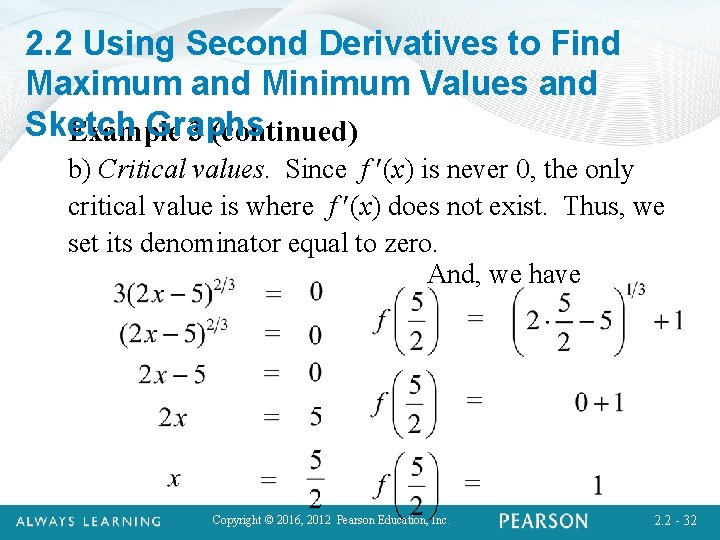2. 2 Using Second Derivatives to Find Maximum and Minimum Values and Sketch Graphs Example 3 (continued) b) Critical values. Since f (x) is never 0, the only critical value is where f (x) does not exist. Thus, we set its denominator equal to zero. And, we have Copyright © 2016, 2012 Pearson Education, Inc. 2. 2 - 32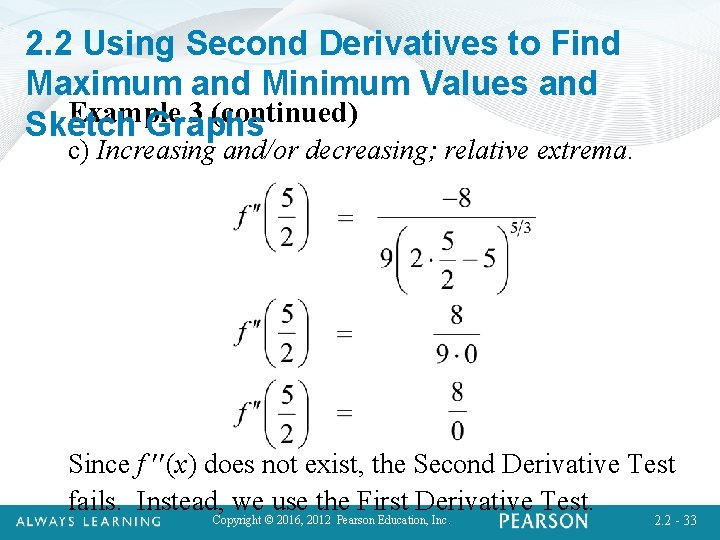2. 2 Using Second Derivatives to Find Maximum and Minimum Values and Example 3 (continued) Sketch Graphs c) Increasing and/or decreasing; relative extrema. Since f (x) does not exist, the Second Derivative Test fails. Instead, Copyright we©use the First Derivative Test. 2016, 2012 Pearson Education, Inc. 2. 2 - 332. 2 Using Second Derivatives to Find Maximum and Minimum Values and Example 3 (continued) Sketch Graphs c) Increasing and/or decreasing; relative extrema (continued). Selecting 2 and 3 as test values on either side of Since f’(x) is positive on both sides of extremum. Copyright © 2016, 2012 Pearson Education, Inc. is not an 2. 2 - 34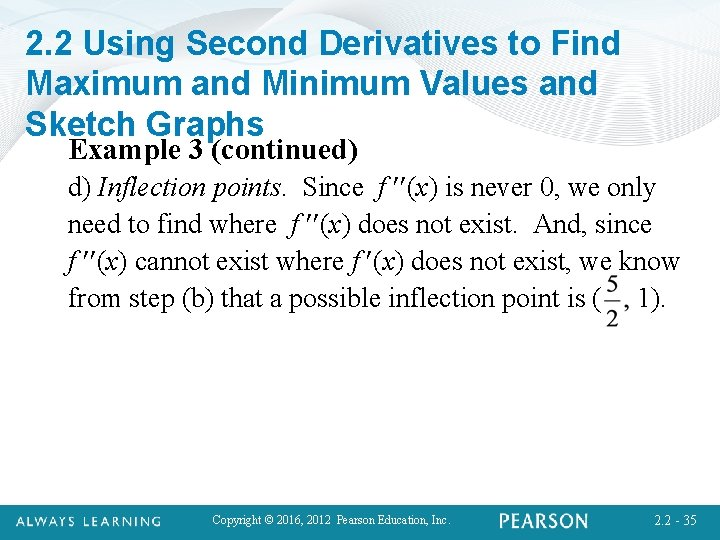2. 2 Using Second Derivatives to Find Maximum and Minimum Values and Sketch Graphs Example 3 (continued) d) Inflection points. Since f (x) is never 0, we only need to find where f (x) does not exist. And, since f (x) cannot exist where f (x) does not exist, we know from step (b) that a possible inflection point is ( 1). Copyright © 2016, 2012 Pearson Education, Inc. 2. 2 - 35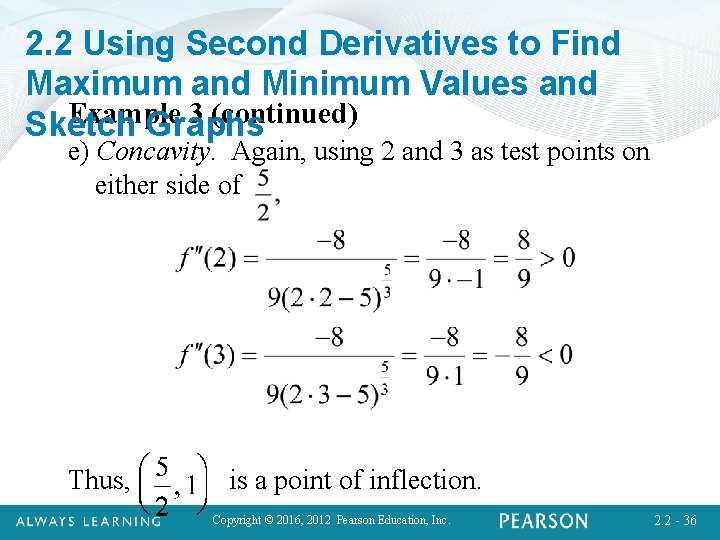2. 2 Using Second Derivatives to Find Maximum and Minimum Values and Example 3 (continued) Sketch Graphs e) Concavity. Again, using 2 and 3 as test points on either side of Thus, is a point of inflection. Copyright © 2016, 2012 Pearson Education, Inc. 2. 2 - 36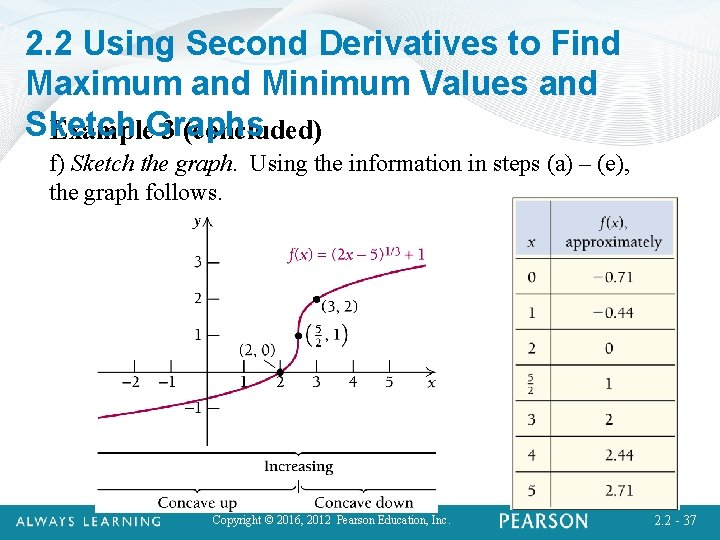2. 2 Using Second Derivatives to Find Maximum and Minimum Values and Sketch Example. Graphs 3 (concluded) f) Sketch the graph. Using the information in steps (a) – (e), the graph follows. Copyright © 2016, 2012 Pearson Education, Inc. 2. 2 - 372. 2 Using Second Derivatives to Find Maximum and Minimum Values and Sketch Graphs Section Summary • The second derivative f is used to determine the concavity of the graph of function f. • If f (x) > 0 for all x in an open interval I, then the graph of f is concave up over I. • If f (x) < 0 for all x in an open interval I, then the graph of f is concave down over I. • If c is a critical value and f (c) > 0, then f (c) is a relative minimum. • If c is a critical value and f (c) < 0, then f (c) is a relative maximum. Copyright © 2016, 2012 Pearson Education, Inc. 2. 2 - 382. 2 Using Second Derivatives to Find Maximum and Minimum Values and Sketch Graphs Section Summary Concluded • If c is a critical value and f (c) = 0, then the First-Derivative Test must be used to determine if f (c) is a relative extrema. • If f (x 0) = 0 or f (x 0) does not exist, and there is a change in concavity to the left and to the right of x 0, then the point ( x 0, f (x 0) ) is called a point of inflection. • Finding relative extrema, intervals over which a function is increasing or decreasing, intervals of upward or downward concavity, and points of inflection is all part of a strategy for accurate curve sketching. Copyright © 2016, 2012 Pearson Education, Inc. 2. 2 - 39